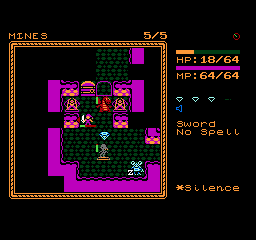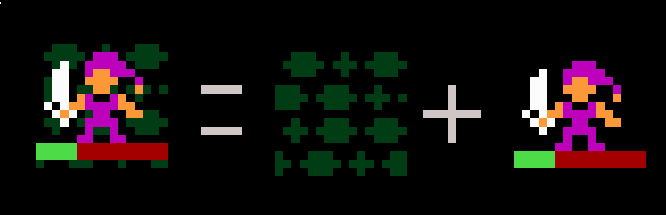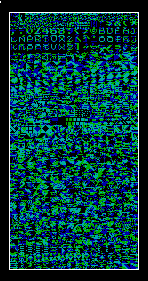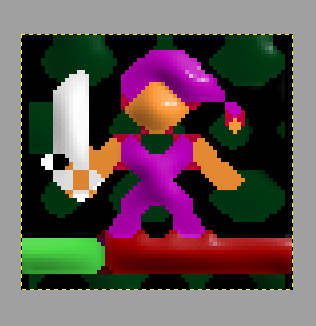# Pixel-art scaling algorithms

We take these filters for granted in our video game emulators. Might be an interesting exercise to port them to G’MIC, esp. for @bazza, @Reptorian and @Joan_Rake1.

1 Like

I’m particularly not so interested into making a pixel-art scaling algorithm at the moment, but it’s a challenge I might take into consideration if I feel like something to do with coding. I’m not sure how to work with matrixes on G’MIC, but I’d be interested into a sample to replicate HIlbert curves.

HQ4x <3

One of the things that always like me that it exist is something that can recognise the sprites real and replace them by new or the best respecting the layer of sprites…

example:withSomething that disarm “collage”. Allowing create the image in HD or with other caracter in his place…

To know as I arm each layer can help us of the titleset:Ok, now that my desktop PC is overheating, I may actually go back to coding G’MIC filter and this is now on my radar. Side note, I wish @Joan_Rake1 is here.As they can separate the planes could create something like this…

I don’t know enough about emulators to know if the filter works on the per layer basis; probably not.

@bazza, I never asked: are you working on an animation or game? If so, do you mind showing some of your work (via a link or something)?I said to do it to graphic level: visual-demosaic

https://bazza.noblogs.org/video-juegos/

1 Like

1 Like

I don’t think that’ll be happening. I wonder if Tensorflow c++ api could be integrated to G’MIC to enable AI G’MIC coding.

There is something you may not realize about neural network methods: the NNs used are huge, composed of billions of coefficients, and usually take several hundred mega or tens of giga in memory to work.
This is not something that can be considered for a software like G’MIC, where filters run on the user’s computer (and not online).
Even if we were to add tensorflow features to G’MIC one day, this would not solve the problem: the neural networks currently described in scientific articles are memory monsters, not at all suitable for integration into mainstream software.

The study of light neural networks with equivalent performance is an open research subject, and few things exist. I think we have to wait a little while until the size of the networks becomes more reasonable before we consider integrating these things into G’MIC.

2 Likes

Well, that’s a downer. On the bright side, computers are getting more efficient each years or that may be too hopeful, so the memory argument gets less relevant over time or that’s just being hopeful, but on the downside, we’re still on the prototype stage for these things to come.

Although my knowledges of programming are not sufficient…
I think that if they divide layers by morphology and upperscale separately could attain good result.

And although tensorflow is very big can use technology like the one of waifu2x

Now that someone mentioned waifu2x, I wonder how would go by translating this to gmic code

``````A B C --\ 1 2
D E F --/ 3 4

1 = B | (A & E & !B & !D)
2 = B | (C & E & !B & !F)
3 = E | (!A & !E & B & D)
4 = E | (!C & !E & B & F)
``````

or this

``````1=P; 2=P; 3=P; 4=P;
IF C==A => 1=A
IF A==B => 2=B
IF D==C => 3=C
IF B==D => 4=D
IF of A, B, C, D, three or more are identical: 1=2=3=4=P
``````

After a sample code, I can get started.

I am finally able to understand how they work if I think of two images where one is scaled and relative position of pixels. I’ll get started on this soon

1 Like

EDIT: Earlier post removed. Finally have everything following the algorithm found in that link.
EDIT: Now removed earlier code at Edit#7 for a refined code of these algorithm.

``````#@cli rep_pr_eagle: (eq. to rep_pixelscale_eagle)
rep_pr_eagle: rep_pixelscale_eagle
#@cli rep_pixelscale_eagle:
rep_pixelscale_eagle:
e[^-1] "Scale Image using EAGLE algorithm"
v -
r2dx 200%,1
f "
id=(x%2+1)+2*(y%2);
S=I(x-2,y-2,0,0,1);
T=I(x,y-2,0,0,1);
U=I(x+2,y-2,0,0,1);
V=I(x-2,y,0,0,1);
C=I(x,y);
W=I(x+2,y,0,0,1);
X=I(x-2,y+2,0,0,1);
Y=I(x,y+2,0,0,1);
Z=I(x+2,y+2,0,0,1);
F=C;
if(id==1,if((V==S)&&(S==T),F=S));
if(id==2,if((T==U)&&(U==W),F=U));
if(id==3,if((V==X)&&(X==Y),F=X));
if(id==4,if((W==Z)&&(Z==Y),F=Z));
F;
"
v +
e[^-1] "Scale Image using AdvMAME2x algorithm"
v -
r2dx 200%,1
f "
id=(x%2+1)+2*(y%2);
C=I(x-2,y,0,0,1);
A=I(x,y-2,0,0,1);
B=I(x+2,y,0,0,1);
D=I(x,y+2,0,0,1);
P=I(x,y);
F=P;
if(id==1,if(C==A&&C!=D&&A!=B,F=A));
if(id==2,if(A==B&&A!=C&&B!=D,F=B));
if(id==3,if(D==C&&D!=B&&C!=A,F=C));
if(id==4,if(B==D&&B!=A&&D!=C,F=D));
F;
"
v +
e[^-1] "Scale Image using AdvMAME3x algorithm"
v -
r2dx 300%,1
f "
id=(x%3+1)+3*(y%3);
A=I(x-3,y-3,0,0,1);
B=I(x,y-3,0,0,1);
C=I(x+3,y-3,0,0,1);
D=I(x-3,y,0,0,1);
E=I(x,y);
F=I(x+3,y,0,0,1);
G=I(x-3,y+3,0,0,1);
H=I(x,y+3,0,0,1);
I=I(x+3,y+3,0,0,1);
Z=E;
if(id==1,if(D==B&&D!=H&&B!=F,Z=D));
if(id==2,if((D==B&&D!=H&&B!=F&&E!=C)||(B==F&&B!=D&&F!=H&&E!=A),Z=B));
if(id==3,if(B==F&&B!=D&&F!=H,Z=F));
if(id==4,if((H==D&&H!=F&&D!=B&&E!=A)||(D==B&&D!=H&&B!=F&&E!=G),Z=D));
if(id==6,if((B==F&&B!=D&&F!=H&&E!=I)||(F==H&&F!=B&&H!=D&&E!=C),Z=F));
if(id==7,if(H==D&&H!=F&&D!=B,Z=D));
if(id==8,if((F==H&&F!=B&&H!=D&&E!=G)||(H==D&&H!=F&&D!=B&&E!=I),Z=H));
if(id==9,if(F==H&&F!=B&&H!=D,Z=F));
Z;
"
v +
``````
1 Like